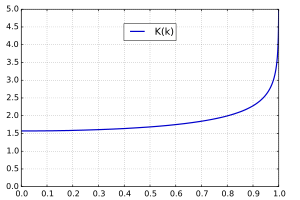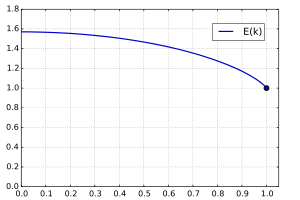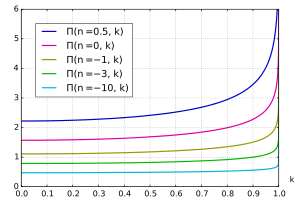# Elliptic integral

In integral calculus, elliptic integrals originally arose in connection with the problem of giving the arc length of an ellipse. They were first studied by Giulio Fagnano and Leonhard Euler. Modern mathematics defines an "elliptic integral" as any function f which can be expressed in the formwhere R is a rational function of its two arguments, P is a polynomial of degree 3 or 4 with no repeated roots, and c is a constant.

In general, integrals in this form cannot be expressed in terms of elementary functions. Exceptions to this general rule are when P has repeated roots, or when R(x,y) contains no odd powers of y. However, with the appropriate reduction formula, every elliptic integral can be brought into a form that involves integrals over rational functions and the three Legendre canonical forms (i.e. the elliptic integrals of the first, second and third kind).

Besides the Legendre form given below, the elliptic integrals may also be expressed in Carlson symmetric form. Additional insight into the theory of the elliptic integral may be gained through the study of the Schwarz–Christoffel mapping. Historically, elliptic functions were discovered as inverse functions of elliptic integrals.

## Argument notation

Incomplete elliptic integrals are functions of two arguments; complete elliptic integrals are functions of a single argument. These arguments are expressed in a variety of different but equivalent ways (they give the same elliptic integral). Most texts adhere to a canonical naming scheme, using the following naming conventions.

For expressing one argument:

Each of the above three quantities is completely determined by any of the others (given that they are non-negative). Thus, they can be used interchangeably.

The other argument can likewise be expressed as φ, the amplitude, or as x or u, where x = sin φ = sn u and sn is one of the Jacobian elliptic functions.

Specifying the value of any one of these quantities determines the others. Note that u also depends on m. Some additional relationships involving u includeThe latter is sometimes called the delta amplitude and written as Δ(φ) = dn u. Sometimes the literature also refers to the complementary parameter, the complementary modulus, or the complementary modular angle. These are further defined in the article on quarter periods.

## Incomplete elliptic integral of the first kind

The incomplete elliptic integral of the first kind F is defined asThis is the trigonometric form of the integral; substituting, one obtains Jacobi's form:Equivalently, in terms of the amplitude and modular angle one has:In this notation, the use of a vertical bar as delimiter indicates that the argument following it is the "parameter" (as defined above), while the backslash indicates that it is the modular angle. The use of a semicolon implies that the argument preceding it is the sine of the amplitude:This potentially confusing use of different argument delimiters is traditional in elliptic integrals and much of the notation is compatible with that used in the reference book by Abramowitz and Stegun and that used in the integral tables by Gradshteyn and Ryzhik.

Withone has:thus, the Jacobian elliptic functions are inverses to the elliptic integrals.

### Notational variants

There are still other conventions for the notation of elliptic integrals employed in the literature. The notation with interchanged arguments, F(k,φ), is often encountered; and similarly E(k,φ) for the integral of the second kind. Abramowitz and Stegun substitute the integral of the first kind, F(φ,k), for the argument φ in their definition of the integrals of the second and third kinds, unless this argument is followed by a backslash: i.e. E(F(φ,k) | k2) for E(φ | k2). Moreover, their complete integrals employ the parameter k2 as argument in place of the modulus k, i.e. K(k2) rather than K(k). And the integral of the third kind defined by Gradshteyn and Ryzhik, Π(φ,n,k), puts the amplitude φ first and not the "characteristic" n.

Thus one must be careful with the notation when using these functions, because various reputable references and software packages use different conventions in the definitions of the elliptic functions. For example, some references, and Wolfram's Mathematica software and Wolfram Alpha, define the complete elliptic integral of the first kind in terms of the parameter m, instead of the elliptic modulus k.## Incomplete elliptic integral of the second kind

The incomplete elliptic integral of the second kind E in trigonometric form isSubstituting, one obtains Jacobi's form:Equivalently, in terms of the amplitude and modular angle:Relations with the Jacobi elliptic functions includeThe meridian arc length from the equator to latitudeis written in terms of E:where a is the semi-major axis, and e is the eccentricity.

## Incomplete elliptic integral of the third kind

The incomplete elliptic integral of the third kind Π isorThe number n is called the characteristic and can take on any value, independently of the other arguments. Note though that the valueis infinite, for any m.

A relation with the Jacobian elliptic functions isThe meridian arc length from the equator to latitudeis also related to a special case of Π:## Complete elliptic integral of the first kindPlot of the complete elliptic integral of the first kindElliptic Integrals are said to be 'complete' when the amplitude φ=π/2 and therefore x=1. The complete elliptic integral of the first kind K may thus be defined asor more compactly in terms of the incomplete integral of the first kind asIt can be expressed as a power serieswhere Pn is the Legendre polynomials, which is equivalent towhere n!! denotes the double factorial. In terms of the Gauss hypergeometric function, the complete elliptic integral of the first kind can be expressed asThe complete elliptic integral of the first kind is sometimes called the quarter period. It can be computed very efficiently in terms of the arithmetic–geometric mean:See Carlson (2010, 19.8) for details.

### Relation to Jacobi θ-function

The relation to Jacobi's θ function is given bywhere the nome q is### Asymptotic expressionsThis approximation has a relative precision better than 3×10−4 for k < 1/2. Keeping only the first two terms is correct to 0.01 precision for k < 1/2. [Citation Needed]

### Differential equation

The differential equation for the elliptic integral of the first kind isA second solution to this equation is. This solution satisfies the relation.

## Complete elliptic integral of the second kindPlot of the complete elliptic integral of the second kindThe complete elliptic integral of the second kind E is defined asor more compactly in terms of the incomplete integral of the second kindasFor an ellipse with semi-major axisand semi-minor axisand eccentricity, the complete elliptic integral of the second kindis equal to one quarter of the circumferenceof the ellipse measured in units of the semi-major axis. In other words:The complete elliptic integral of the second kind can be expressed as a power serieswhich is equivalent toIn terms of the Gauss hypergeometric function, the complete elliptic integral of the second kind can be expressed asThe complete elliptic integral of the second kind can also be computed very efficiently using the arithmetic–geometric mean (Carlson 2010, 19.8).

### Derivative and differential equationA second solution to this equation is.

## Complete elliptic integral of the third kindPlot of the complete elliptic integral of the third kindwith several fixed values ofThe complete elliptic integral of the third kind Π can be defined asNote that sometimes the elliptic integral of the third kind is defined with an inverse sign for the characteristic n,Just like the complete elliptic integrals of the first and second kind, the complete elliptic integral of the third kind can be computed very efficiently using the arithmetic-geometric mean (Carlson 2010, 19.8).

### Partial derivatives## Functional relationsWikimedia Commons has media related to Elliptic integral.